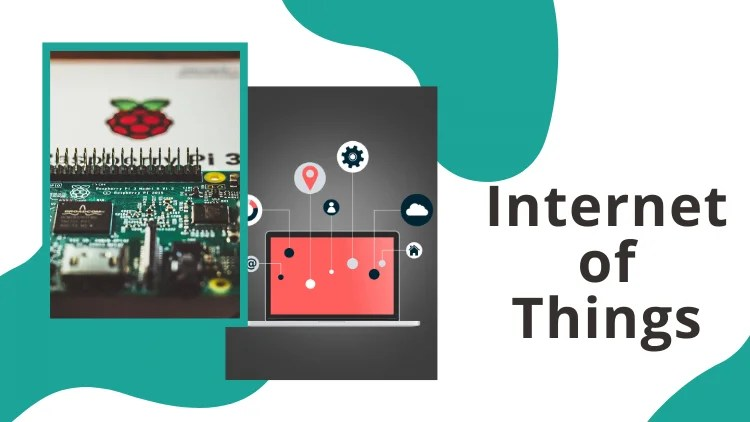Select Page

This is a video tutorial about how to interface raspberry Pi with ultrasonic sensor. Ultrasonic sensor is used for distance measurement and gives fairly accurate readings as its using ultrasonic sound to find the distance. This is a detailed tutorial including all the explanation about interfacing of sensor, its working, and the algorithm of python program.

The python program is also explained in detail in the video.

To interface raspberry pi with ultrasonic sensor and create a distance meter, you’ll need

• Raspberry Pi any version
• Ultrasonic sensor HC-SR04
• 1k Resistor
• 1.5k Resistor
• Connecting Wires

## Circuit Diagram of Ultrasonic sensor with Raspberry Pi

The interfacing diagram is as shown below

## Python Code for Ultrasonic Sensor Interfacing with Raspberry Pi

```import RPi.GPIO as GPIO
import time
TRIG=21
ECHO=20
GPIO.setmode(GPIO.BCM)
while True:
print"distance measurement in progress"
GPIO.setup(TRIG,GPIO.OUT)
GPIO.setup(ECHO,GPIO.IN)
GPIO.output(TRIG,False)
print"waiting for sensor to settle"
time.sleep(0.2)
GPIO.output(TRIG,True)
time.sleep(0.00001)
GPIO.output(TRIG,False)
while GPIO.input(ECHO)==0:
pulse_start=time.time()
while GPIO.input(ECHO)==1:
pulse_end=time.time()
pulse_duration=pulse_end-pulse_start
distance=pulse_duration*17150
distance=round(distance,2)
print"distance:",distance,"cm"
time.sleep(2)
```

Above code is created in Python 3, however if you’re using python 2 the code will still work for you.

## Complete Video tutorial

This is the detailed tutorial of Ultrasonic Sensor Interfacing with Raspberry Pi

Keep experimenting!!!